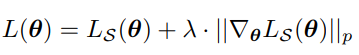# Add_(): argument 'other' (position 1) must be Tensor, not list

I’m trying to implement SGD for which I want to penalize the gradient norm resulting in the following loss function. The reason for this is that it has been assosicated with leading to flat minima in deep learning. The loss function looks like this:This derives from the paper  for which my implementation was inspired by .

I started the implementation this for SGD but have the problem that the computed I do not know how I to pass the newly computed parameters to my inplace operator `p.data.add_` since the newly computed parameters are not tensors but a list. I want to stack the parameters (not concatenate the parameters) together but since they are of different dimension I do not know how to go about this.

I would be happy for guidance.

Here is my code:

``````import numpy as np
import torch
from torch.optim import Optimizer
import optree
from torch.optim.optimizer import Optimizer, required
from typing import Optional, List

class SGD(Optimizer):

@dataclass
class State:
momentum: np.ndarray

def __init__(self,
params,
lr=required,
momentum=0,
dampening=0,
weight_decay=0,
nesterov=False,
maximize=False,
foreach: Optional[bool] = None,
differentiable = False,
):

if lr is not required and lr < 0.0:
raise ValueError("Invalid learning rate: {}".format(lr))
if momentum < 0.0:
raise ValueError("Invalid momentum value: {}".format(momentum))
if weight_decay < 0.0:
raise ValueError("Invalid weight_decay value: {}".format(weight_decay))

defaults = dict(lr=lr, momentum=momentum, dampening=dampening,
weight_decay=weight_decay, nesterov=nesterov,
maximize=maximize, foreach=foreach,
differentiable=differentiable,
if nesterov and (momentum <= 0 or dampening != 0):
raise ValueError("Nesterov momentum requires a momentum and zero dampening")
super(SGD, self).__init__(params, defaults)

def __setstate__(self, state):
super().__setstate__(state)
for group in self.param_groups:
group.setdefault('nesterov', False)
group.setdefault('maximize', False)
group.setdefault('foreach', None)
group.setdefault('differentiable', False)
group.setdefault('lr', 0.001)

def step(self, closure=None):
loss = None
if closure is not None:
loss = closure()

print(self.param_groups['params'])

d_p_list = []
momentum_buffer_list = []
for group in self.param_groups:

weight_decay = group['weight_decay']
momentum = group['momentum']
dampening = group['dampening']
nesterov = group['nesterov']

for p in group['params']:

state = self.state[p]
if 'momentum_buffer' not in state:
momentum_buffer_list.append(None)
else:
momentum_buffer_list.append(state['momentum_buffer'])

states = list(self.state.values())
params_flat, treedef = optree.tree_flatten(d_p_list)
states_flat = treedef.flatten_up_to(states)

out = [
]

new_params_flat, new_states_flat = list(zip(*out)) if out else ((), ())
new_param = optree.tree_unflatten(treedef, new_params_flat)
new_states = optree.tree_unflatten(treedef, new_states_flat)

new_param = [nn.Parameter(param) for param in new_param]

self.state = new_states
#self.state[p] = new_state
return loss

momentum_buffer_list = []

d_p = params.clone()
for i, param in enumerate(params):
if hyper_params['weight_decay'] != 0:

if hyper_params['momentum'] != 0:
buf = momentum_buffer_list[i]

if buf is None:
buf = torch.clone(param).detach()
momentum_buffer_list[i] = buf
else:

if hyper_params['nesterov']:
else:
d_p = buf

new_param = param - hyper_params['lr'] * d_p
new_state = SGD.State(new_param)

return new_param, new_state

if __name__ == '__main__':
from torch import nn
model = nn.Linear(10, 20)
optimizer = SGD(model.parameters(), lr=1e-3)
#optimizer.step()
# Create dummy backward pass
m = model(torch.randn(1, 10))
m.mean().backward()
optimizer.step()
``````````

This won’t work since PyTorch expects the parameters to be tensors.
Even if you could replace a single trainable parameter with a `list`, could you describe how this parameter should be used in the next forward pass (e.g. the `weight` parameter of an `nn.Linear` layer)?

Hello ptrblck,

thank you for your response and your time. According to the paper the idea of penalizing the gradient norm is adaptable to any optimizer. I chose to SGD in this example. The forward pass therefore is handled as a usual (can be seen when replacing `p.data.add_(torch.hstack(new_param))` with `p.data.add_(torch.hstack(d_p_list))` (old parameters) where the only problem is the data type. Is there a work around for resolving the dtype issue?

Could you describe the data type error you are seeing or post the actual error message?
If you are running into a `dtype` mismatch I would assume transforming the stacked tensor should work e.g. via:

``````with torch.no_grad():